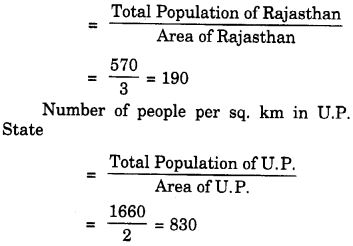NCERT Solutions for Class 7 Maths Chapter 8 Comparing Quantities Ex 8.1 are part of NCERT Solutions for Class 7 Maths. Here we have given NCERT Solutions for Class 7 Maths Chapter 8 Comparing Quantities Ex 8.1.

 Board CBSE Textbook NCERT Class Class 7 Subject Maths Chapter Chapter 8 Chapter Name Comparing Quantities Exercise Ex 8.1 Number of Questions Solved 3 Category NCERT Solutions

## NCERT Solutions for Class 7 Maths Chapter 8 Comparing Quantities Ex 8.1

Question 1.
Find the ratio of:
(a) ₹ 5 to 50 paise
(b) 15 kg to 210 g
(c) 9 m to 27 cm
(d) 30 days to 36 hour

Solution:
(a) ₹ 5 : 50 paise
= ₹ 5 : 50 paise
= 5 × 100 paise : 50 paise
= 500 paise : 50 paise
= 10 : 1.

(b) 15 kg to 210g
= 15 kg : 210g
= 15 × 1000 g : 210g
= 15000 g : 210g = 500:7.

(c) 9 m to 27 cm
= 9m : 27 cm = 9 × 100 cm : 27 cm
= 900 cm : 27 cm
900 : 27 = 100 : 3.

(d) 30 days to 36 hours
= 30 days : 36 hours
30 × 24 hours : 36 hours
= 720 hours : 36 hours
= 720 : 36 = 20 : 1.

Question 2.
In a computer lab, there are 3 computers for every 6 students. How many computers will be needed for 24 students?
Solution:
Let x computers be needed for 24 students.Hence, 12 computers will be needed for
24 students.
Aliter:
In a computer lab,
Every 6 students need 3 computers.
∴ Every 1 student needs $$\frac { 3 }{ 6 }$$ computers.
∴ Every 24 students need $$\frac { 3 }{ 6 }$$ × 24 = 3 × 4 = 12 computers

Question 3.
Population of Rajasthan = 570 lakhs
and population of UP = 1660 lakhs.
Area of Rajasthan =3 lakh km2
and area of UP = 2 lakh km2.
(i) How many people are there per km2 in both these States?
(ii) Which State is less populated?
Solution:
(i) Number of people per sq. km. in Rajasthan state(ii) The Rajasthan State is less populated.

We hope the NCERT Solutions for Class 7 Maths Chapter 8 Comparing Quantities Ex 8.1 helps you. If you have any query regarding NCERT Solutions for Class 7 Maths Chapter 8 Comparing Quantities Ex 8.1, drop a comment below and we will get back to you at the earliest.